# Introduction to tensor slicing

When working on ML applications such as object detection and NLP, it is sometimes necessary to work with sub-sections (slices) of tensors. For example, if your model architecture includes routing, where one layer might control which training example gets routed to the next layer. In this case, you could use tensor slicing ops to split the tensors up and put them back together in the right order.

In NLP applications, you can use tensor slicing to perform word masking while training. For example, you can generate training data from a list of sentences by choosing a word index to mask in each sentence, taking the word out as a label, and then replacing the chosen word with a mask token.

In this guide, you will learn how to use the TensorFlow APIs to:

• Extract slices from a tensor
• Insert data at specific indices in a tensor

This guide assumes familiarity with tensor indexing. Read the indexing sections of the Tensor and TensorFlow NumPy guides before getting started with this guide.

## Setup

``````import tensorflow as tf
import numpy as np
``````

## Extract tensor slices

Perform NumPy-like tensor slicing using `tf.slice`.

``````t1 = tf.constant([0, 1, 2, 3, 4, 5, 6, 7])

print(tf.slice(t1,
begin=,
size=))
``````

Alternatively, you can use a more Pythonic syntax. Note that tensor slices are evenly spaced over a start-stop range.

``````print(t1[1:4])
``````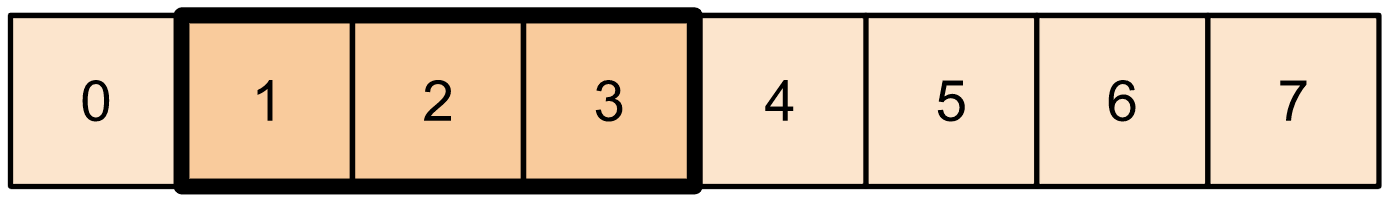``````print(t1[-3:])
``````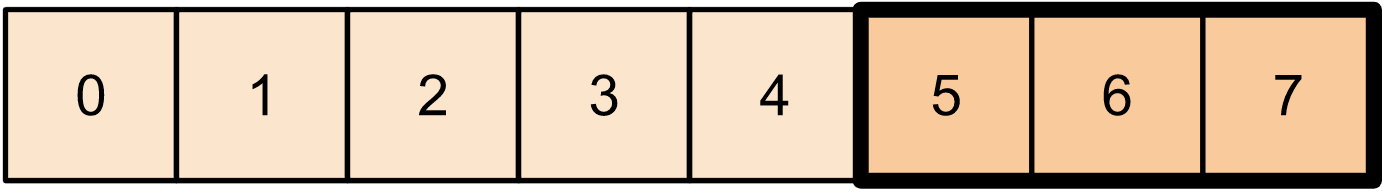For 2-dimensional tensors,you can use something like:

``````t2 = tf.constant([[0, 1, 2, 3, 4],
[5, 6, 7, 8, 9],
[10, 11, 12, 13, 14],
[15, 16, 17, 18, 19]])

print(t2[:-1, 1:3])
``````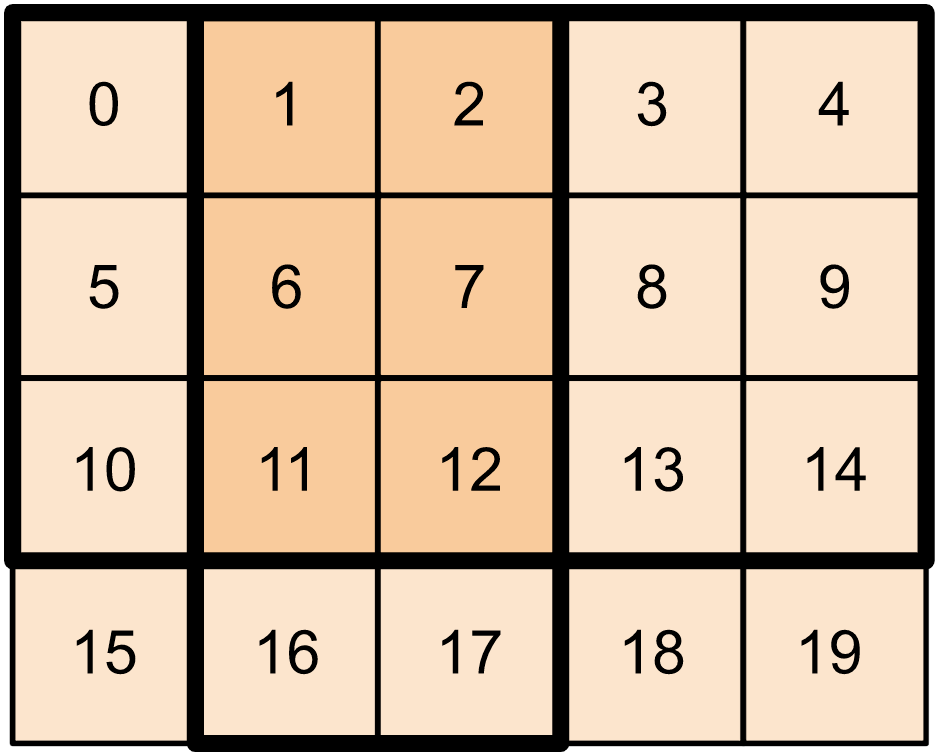You can use `tf.slice` on higher dimensional tensors as well.

``````t3 = tf.constant([[[1, 3, 5, 7],
[9, 11, 13, 15]],
[[17, 19, 21, 23],
[25, 27, 29, 31]]
])

print(tf.slice(t3,
begin=[1, 1, 0],
size=[1, 1, 2]))
``````

You can also use `tf.strided_slice` to extract slices of tensors by 'striding' over the tensor dimensions.

Use `tf.gather` to extract specific indices from a single axis of a tensor.

``````print(tf.gather(t1,
indices=[0, 3, 6]))

# This is similar to doing

t1[::3]
```````tf.gather` does not require indices to be evenly spaced.

``````alphabet = tf.constant(list('abcdefghijklmnopqrstuvwxyz'))

print(tf.gather(alphabet,
indices=[2, 0, 19, 18]))
``````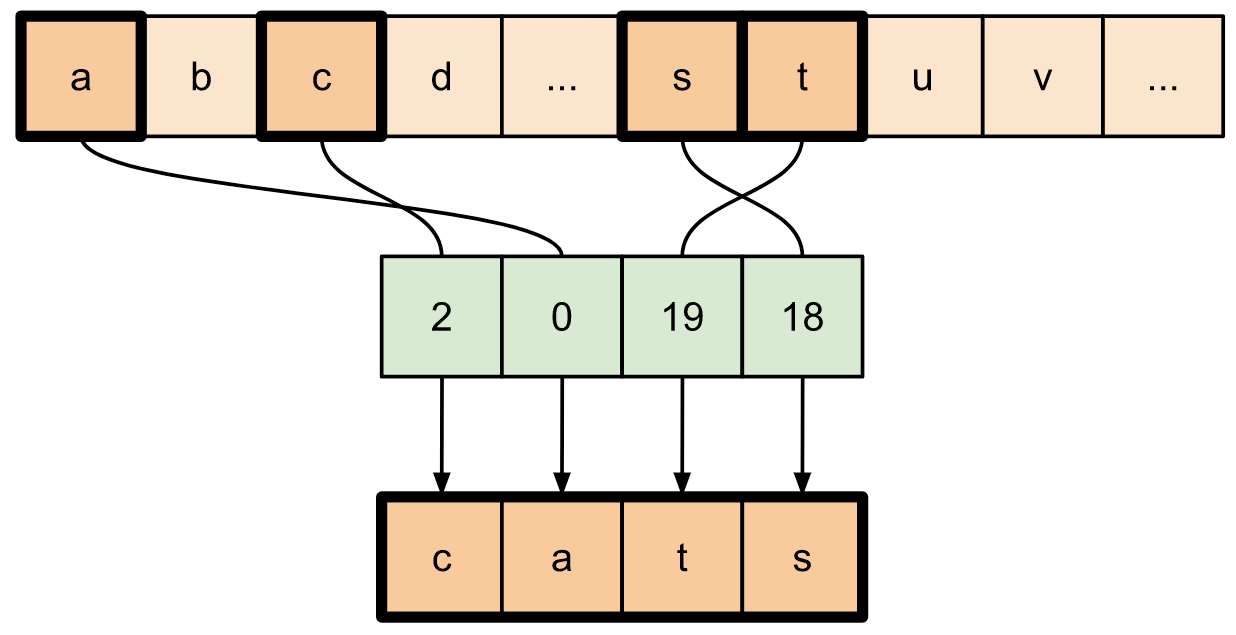To extract slices from multiple axes of a tensor, use `tf.gather_nd`. This is useful when you want to gather the elements of a matrix as opposed to just its rows or columns.

``````t4 = tf.constant([[0, 5],
[1, 6],
[2, 7],
[3, 8],
[4, 9]])

print(tf.gather_nd(t4,
indices=[, , ]))
``````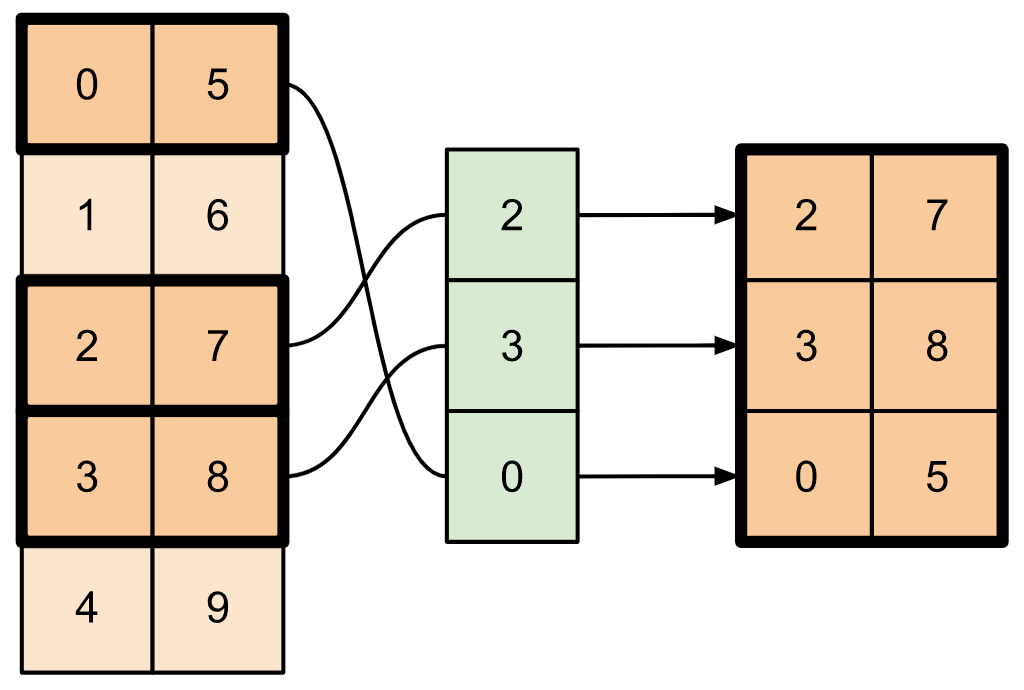``````t5 = np.reshape(np.arange(18), [2, 3, 3])

print(tf.gather_nd(t5,
indices=[[0, 0, 0], [1, 2, 1]]))
``````
``````# Return a list of two matrices

print(tf.gather_nd(t5,
indices=[[[0, 0], [0, 2]], [[1, 0], [1, 2]]]))
``````
``````# Return one matrix

print(tf.gather_nd(t5,
indices=[[0, 0], [0, 2], [1, 0], [1, 2]]))
``````

## Insert data into tensors

Use `tf.scatter_nd` to insert data at specific slices/indices of a tensor. Note that the tensor into which you insert values is zero-initialized.

``````t6 = tf.constant()
indices = tf.constant([, , , , ])
data = tf.constant([2, 4, 6, 8, 10])

print(tf.scatter_nd(indices=indices,
shape=t6))
``````

Methods like `tf.scatter_nd` which require zero-initialized tensors are similar to sparse tensor initializers. You can use `tf.gather_nd` and `tf.scatter_nd` to mimic the behavior of sparse tensor ops.

Consider an example where you construct a sparse tensor using these two methods in conjunction.

``````# Gather values from one tensor by specifying indices

new_indices = tf.constant([[0, 2], [2, 1], [3, 3]])
t7 = tf.gather_nd(t2, indices=new_indices)
``````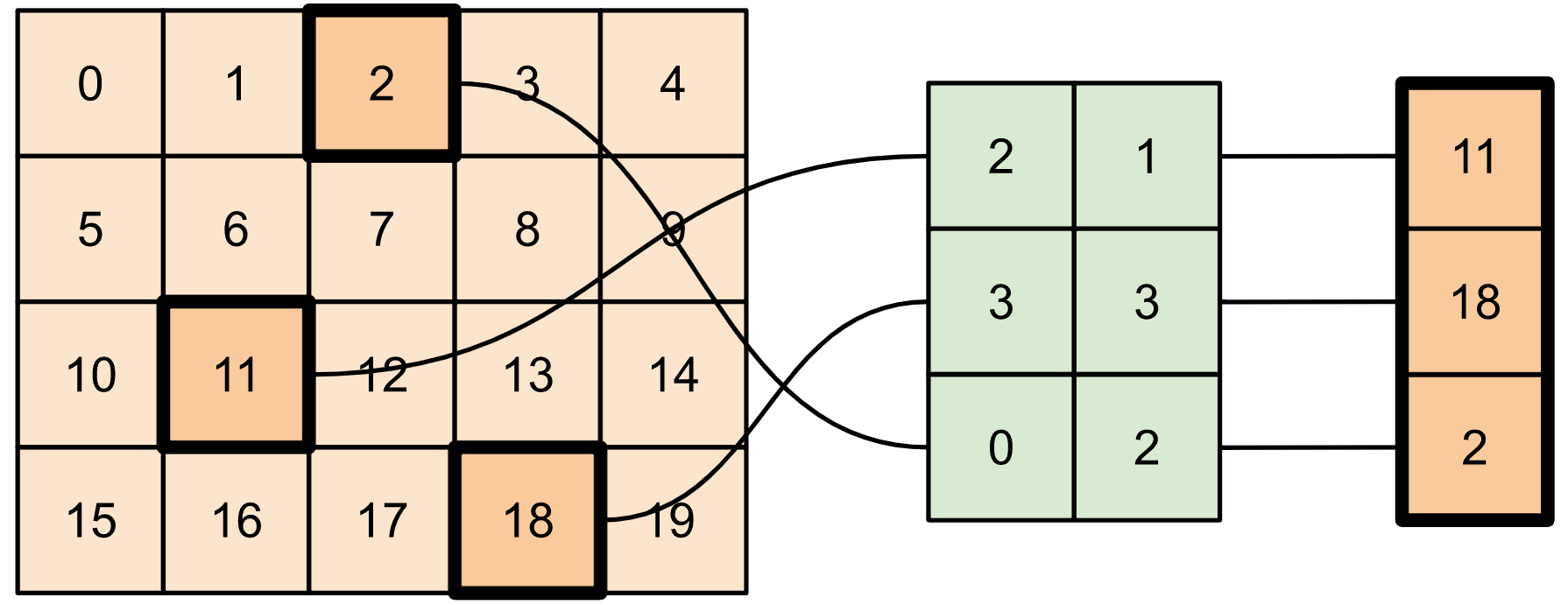``````# Add these values into a new tensor

t8 = tf.scatter_nd(indices=new_indices, updates=t7, shape=tf.constant([4, 5]))

print(t8)
``````

This is similar to:

``````t9 = tf.SparseTensor(indices=[[0, 2], [2, 1], [3, 3]],
values=[2, 11, 18],
dense_shape=[4, 5])

print(t9)
``````
``````# Convert the sparse tensor into a dense tensor

t10 = tf.sparse.to_dense(t9)

print(t10)
``````

To insert data into a tensor with pre-existing values, use `tf.tensor_scatter_nd_add`.

``````t11 = tf.constant([[2, 7, 0],
[9, 0, 1],
[0, 3, 8]])

# Convert the tensor into a magic square by inserting numbers at appropriate indices

indices=[[0, 2], [1, 1], [2, 0]],

print(t12)
``````

Similarly, use `tf.tensor_scatter_nd_sub` to subtract values from a tensor with pre-existing values.

``````# Convert the tensor into an identity matrix

t13 = tf.tensor_scatter_nd_sub(t11,
indices=[[0, 0], [0, 1], [1, 0], [1, 1], [1, 2], [2, 1], [2, 2]],
updates=[1, 7, 9, -1, 1, 3, 7])

print(t13)
``````

Use `tf.tensor_scatter_nd_min` to copy element-wise minimum values from one tensor to another.

``````t14 = tf.constant([[-2, -7, 0],
[-9, 0, 1],
[0, -3, -8]])

t15 = tf.tensor_scatter_nd_min(t14,
indices=[[0, 2], [1, 1], [2, 0]],

print(t15)
``````

Similarly, use `tf.tensor_scatter_nd_max` to copy element-wise maximum values from one tensor to another.

``````t16 = tf.tensor_scatter_nd_max(t14,
indices=[[0, 2], [1, 1], [2, 0]],